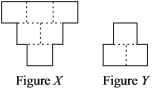# User Forum

Subject :IMO    Class : Class 6

Figure X is made up of 6 identical squares. Three squares were removed from figure X to form figure Y. The perimeter of figure X is 300 m. What is the perimeter of figure Y?A150 m
B200 m
C250 m
D300 m

how

## Ans 1:

Class : Class 9
the ans. is 200m . fig x has 12 sides so length of 1 side = 300/12 =25m . fig y has 8 sides so perimeter = 25 x 8 = 200m

Class : Class 10

Class : Class 6
iu7tgo87rfo

Class : Class 6
B